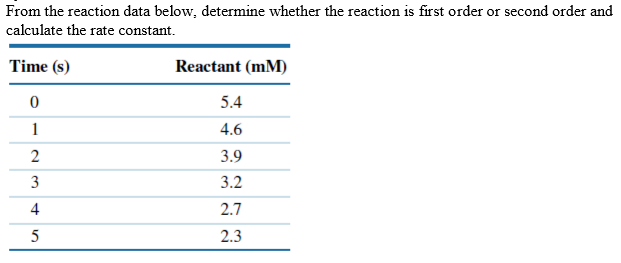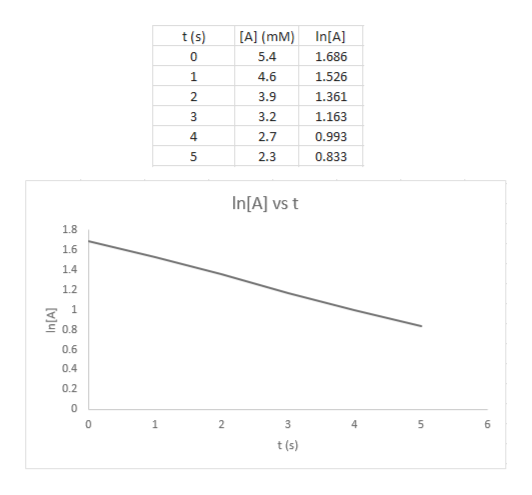# From the reaction data below, determine whether the reaction is first order or second order andcalculate the rate constant.Time (s)Reactant (mM)05.414.623.933.22.72.35

Question
28 views

From the reaction data below, determine whether the reaction is first-order or second-order and calculate the rate constant. See the table in the image.help_outlineImage TranscriptioncloseFrom the reaction data below, determine whether the reaction is first order or second order and calculate the rate constant. Time (s) Reactant (mM) 0 5.4 1 4.6 2 3.9 3 3.2 2.7 2.3 5 fullscreen
check_circle

Step 1

The integrated rate law for zero-order (1), first-order (2), and second-order (3) reaction are given below as equation in which [A] is the concentration of reactant at time t, [A]o is the initial concentration of the reactant, and k is rate constant of the reaction.

Step 2

The graphs, [A] vs t, ln[A] vs t, and 1/[A] vs t are plotted. It is found that the graph between ln[A] and t is a straight line. A first-order reaction gives ...help_outlineImage TranscriptioncloseIn[A A] (mM) t(s) 5.4 1.686 1 4.6 1.526 2. 3.9 1.361 3.2 1.163 2.7 4 0.993 0.833 5 2.3 In[A] vs t 1.8 1.6 14 1.2 1 0.8 0.6 0.4 0.2 0 1 2 6 t(s) un t fullscreen

### Want to see the full answer?

See Solution

#### Want to see this answer and more?

Solutions are written by subject experts who are available 24/7. Questions are typically answered within 1 hour.*

See Solution
*Response times may vary by subject and question.
Tagged in

### Chemical Kinetics